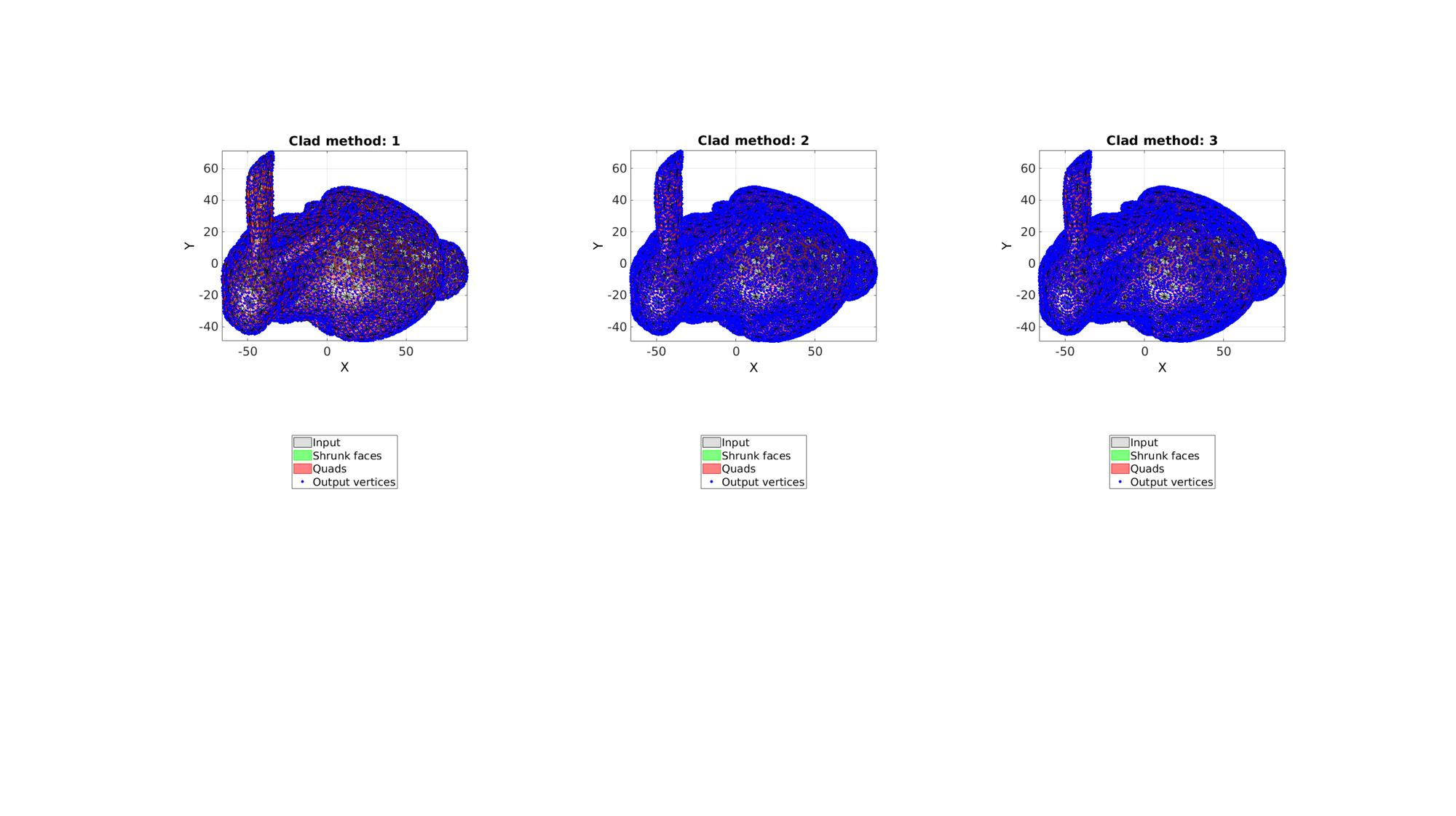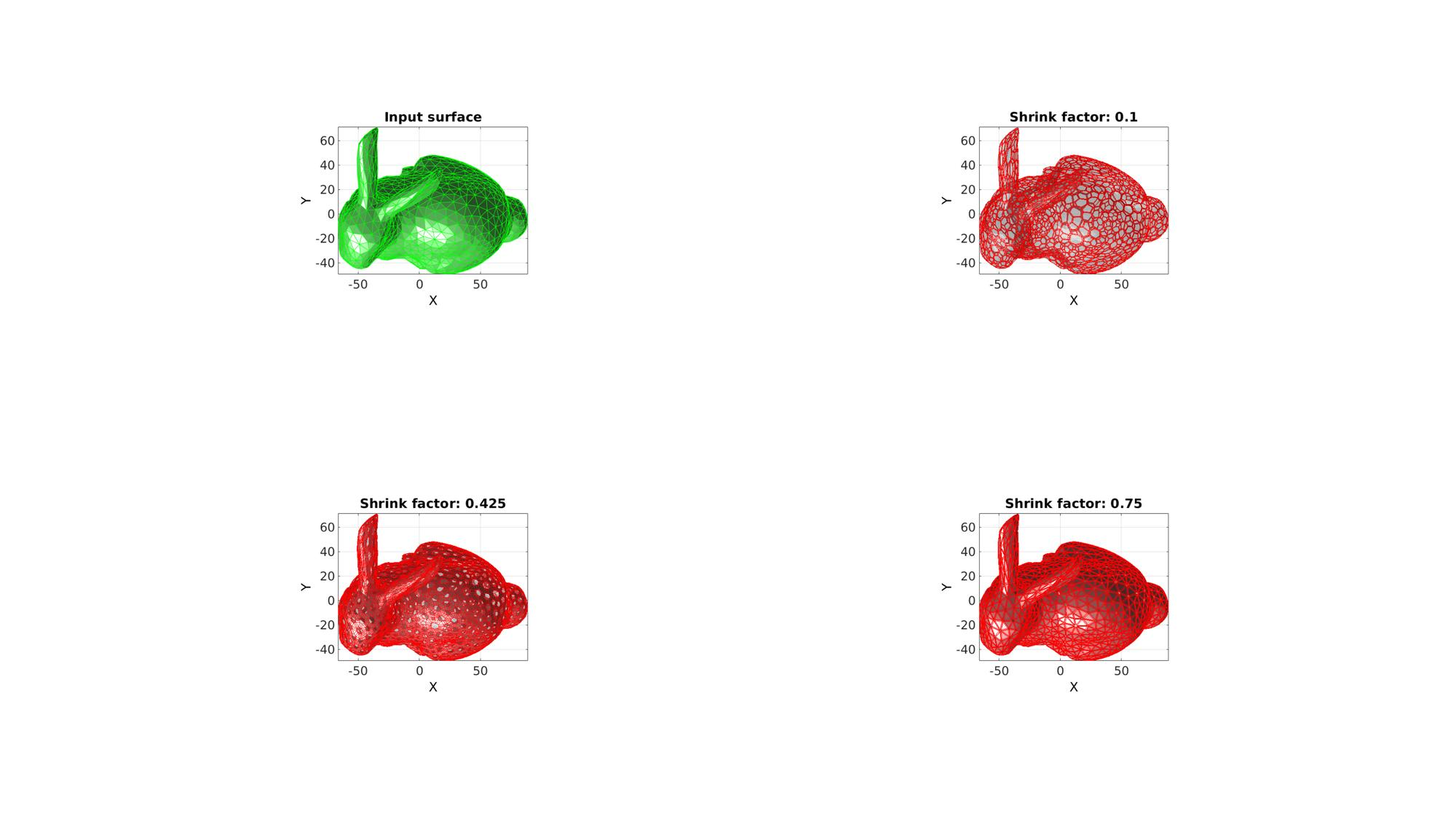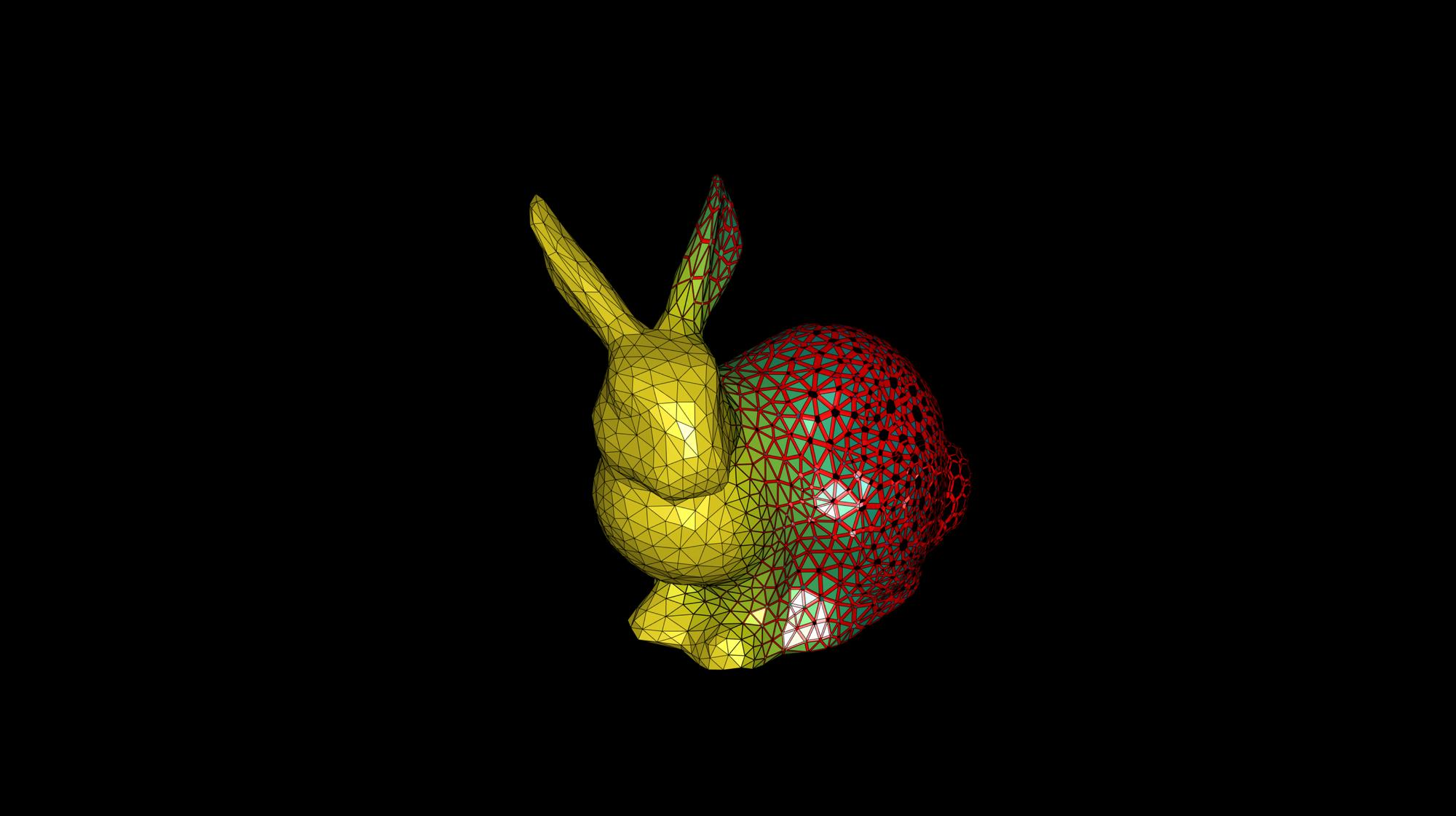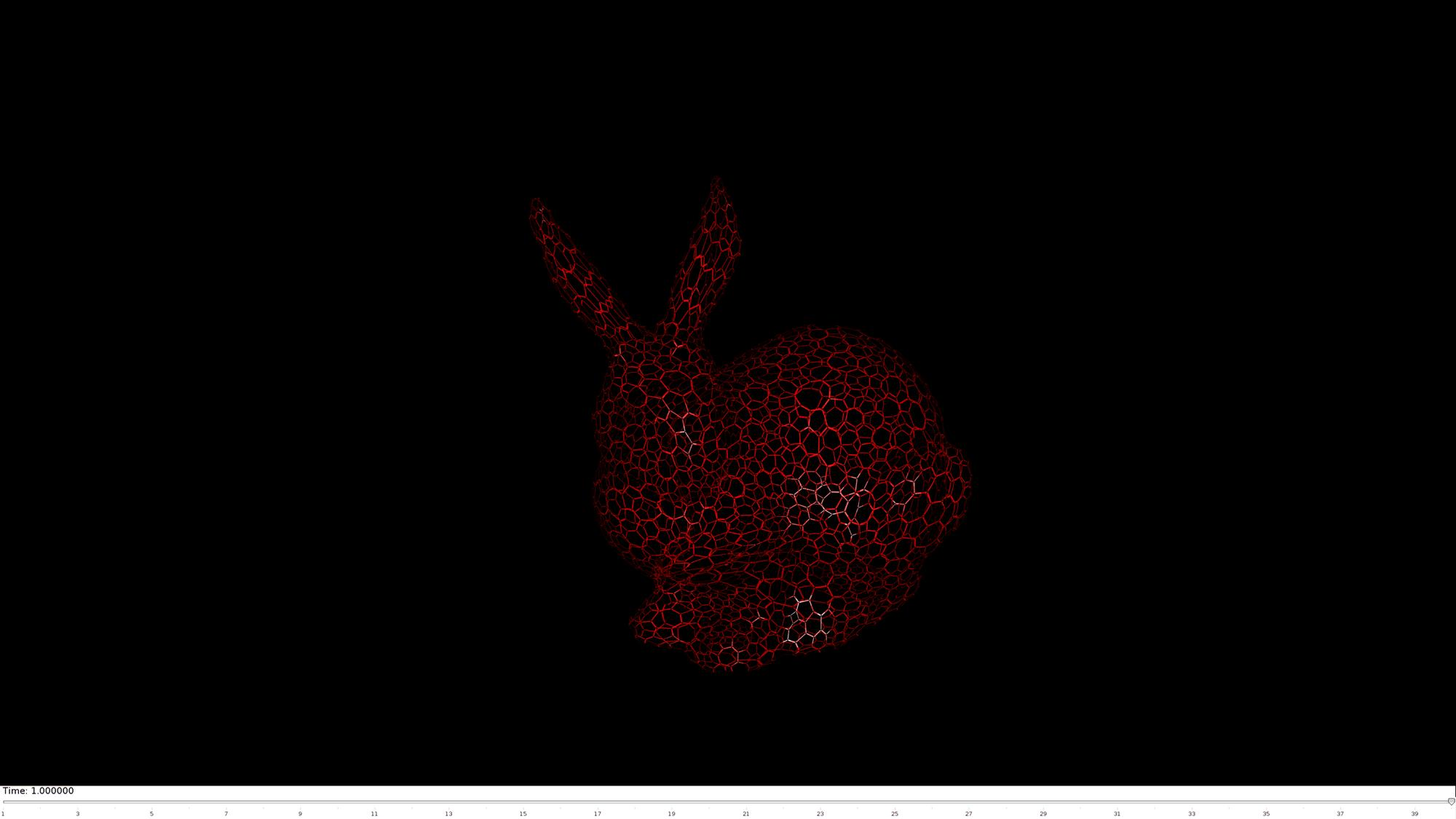Below is a demonstration of the features of the dualClad function

## Description

This function creates patch data defining a cladding on a surface. The cladding is obtained by shrinking the input faces around their mean and by connecting the face sides to form new faces. The output faces cover the edges of the dual of the input surface (hence the name). The output is the set of shrunk faces (same type as input) and a set of new quadrilateral faces connecting the shrunk faces. The shrink factor, which can be a constant or a spatially varying metric on the nodes or faces, defines the face and edge shrink used. The clad method determines whether the output mesh is connected: 1: From shrunk face to shrunk face 2: From shrunk face to shrunk edge to shrunk face 3: From shrunk face to shrunk face through edge (computes intersection at edge which may not be the centre of edge. This method avoids potential "kinks" seen for method 2.

## Examples

```clear; close all; clc;
```

Plot settings

```figStruct.ColorDef='black';
figStruct.Color='k';
```

Specify test surface. Alter settings to test for different geometries and surface types.

```%Testing settings
testCase=2; %1= sphere, 2=bunny, 3=dino
cutMesh=0; %0=not cut, 1=cut in half

switch testCase
case 1
[F,V,~]=geoSphere(2,1); % Building a geodesic dome surface model
shrinkFactor=0.25;
case 2
[F,V]=stanford_bunny('g'); %Bunny
V_mean=mean(V,1);
V=V-V_mean(ones(size(V,1),1),:);
shrinkFactor=0.25;
case 3
[F,V]=parasaurolophus; %dino
V_mean=mean(V,1);
V=V-V_mean(ones(size(V,1),1),:);
shrinkFactor=0.25;
case 4
defaultFolder = fileparts(fileparts(mfilename('fullpath')));
pathName=fullfile(defaultFolder,'data','libSurf');

F=dataStruct.F;
V=dataStruct.V;
shrinkFactor=0.5;
case 5
defaultFolder = fileparts(fileparts(mfilename('fullpath')));
pathName=fullfile(defaultFolder,'data','libSurf');

F=dataStruct.F;
V=dataStruct.V;
shrinkFactor=0.5;
end

if meshType==2
end

if cutMesh==1
logicKeep=V(:,1)<mean(V(:,1));
logicKeep=all(logicKeep(F),2);
F=F(logicKeep,:);
[F,V]=patchCleanUnused(F,V);
end
```

## Example 1: Explaining the clad method

```cladMethods=[1 2 3]; %1= fact-to-face connections, 2=
```

Visualize results

```cFigure;

subplot(1,3,q); hold on;
h(1)=gpatch(F,V,'kw','k',0.25);
h(2)=gpatch(Fc,Vc,'gw','g',1);
h(3)=gpatch(Fq,Vq,'rw','r',1);
h(4)=plotV([Vc;Vq],'b.','MarkerSize',15);
axisGeom;
view(2);
hl.Location='SouthOutside';
end

drawnow;
```## Example 2: Explaining the shrink factor

```cladMethod=3; %1= fact-to-face connections, 2=

shrinkFactors=linspace(0.1,0.75,3); %A range of shrink factors
```

Visualize results

```cFigure;
subplot(2,2,1); hold on;
title('Input surface');
gpatch(F,V,'gw','g',1);

axisGeom;
view(2);

for q=1:1:numel(shrinkFactors)

subplot(2,2,q+1); hold on;
title(['Shrink factor: ',num2str(shrinkFactors(q))]);
gpatch(Fc,Vc,'rw','r',1);
gpatch(Fq,Vq,'rw','r',1);
gpatch(F,V,'kw','none',0.25);
axisGeom;
view(2);
end

drawnow;
```## Example 3: Spatially varying shrink factors

An animation will be created to show effect of a spatially varying shrink factor

```cladMethod=3;

% Define spatially varying shrink factor
shrinkFactor=-V(:,1);
shrinkFactor=mean(shrinkFactor(F),2);
shrinkFactor=shrinkFactor-min(shrinkFactor(:));
shrinkFactor=shrinkFactor./max(shrinkFactor(:));
shrinkFactor=shrinkFactor*1.2;
shrinkFactor=shrinkFactor+0.05;
shrinkFactor(shrinkFactor>1)=1;

```

Animating the effect of the shrink factor

Initialize scene

```hf=cFigure(figStruct);
hold on;

hp1=gpatch(Fc,Vc,shrinkFactor,'k',1);
hp2=gpatch(Fq,Vq,'r','none',1);

axisGeom;
colormap(viridis(250));
caxis([0 1]);
drawnow;
axis off
axis manual;
```Animate scene

```nSteps=40; %Number of animation steps
animStruct.Time=linspace(0,1,nSteps); %Create the time vector

t=linspace(0,2,nSteps);
shrinkFactor=-V(:,1);
shrinkFactor=mean(shrinkFactor(F),2);
shrinkFactor=shrinkFactor-min(shrinkFactor(:));
shrinkFactor=shrinkFactor./max(shrinkFactor(:));
shrinkFactor=shrinkFactor+1;
minLevel=0.05;

for q=1:1:nSteps

shrinkFactorNow=shrinkFactor;
shrinkFactorNow=shrinkFactorNow-t(q);
shrinkFactorNow(shrinkFactorNow<minLevel)=minLevel;
shrinkFactorNow(shrinkFactorNow>1)=1;

%Set entries in animation structure
animStruct.Handles{q}=[hp1 hp2 hp1]; %Handles of objects to animate
animStruct.Props{q}={'Vertices','Vertices','CData'}; %Properties of objects to animate
animStruct.Set{q}={Vc,Vq,shrinkFactorNow}; %Property values for to set in order to animate
end

anim8(hf,animStruct); %Initiate animation
```GIBBON www.gibboncode.org

Kevin Mattheus Moerman, [email protected]

GIBBON footer text

GIBBON: The Geometry and Image-based Bioengineering add-On. A toolbox for image segmentation, image-based modeling, meshing, and finite element analysis.

Copyright (C) 2019 Kevin Mattheus Moerman

This program is free software: you can redistribute it and/or modify it under the terms of the GNU General Public License as published by the Free Software Foundation, either version 3 of the License, or (at your option) any later version.

This program is distributed in the hope that it will be useful, but WITHOUT ANY WARRANTY; without even the implied warranty of MERCHANTABILITY or FITNESS FOR A PARTICULAR PURPOSE. See the GNU General Public License for more details.

You should have received a copy of the GNU General Public License along with this program. If not, see http://www.gnu.org/licenses/.